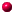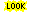´

# PERMUTATION Objects in SYMMETRICA

Inside Symmetrica there are many routines, which are working with the symmetric groups, so it is clear that permutations are fundamental data type.

there are several methods of implementation. Like in the general case there is a tag on the PERMUTATION object, which allows to recognize the special typ of permutation.

a incomplete collection of routines, which work with PERMUTATION objects.copyRothe diagramminverslehmercodem_part_perm = to generate a permutation of given cycle type.multnextobjectread/ objectwrite = I/O in a fileprint/println/fprint/fprintlnrandom_permutationrank_permutationrz = reduced decompositionscan = input of a PERMUTATION objecttex = TeX outputzykeltyp...